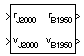# Julian Epoch to Besselian Epoch

Transform position and velocity components from Standard Julian Epoch (J2000) to discontinued Standard Besselian Epoch (B1950)

• Library:
• Aerospace Blockset / Utilities / Axes Transformations

•## Description

The Julian Epoch to Besselian Epoch block transforms two 3-by-1 vectors of Julian Epoch position $\left({\overline{r}}_{J2000}\right)$, and Julian Epoch velocity $\left({\overline{v}}_{J2000}\right)$ into Besselian Epoch position $\left({\overline{r}}_{B1950}\right)$, and Besselian Epoch velocity $\left({\overline{v}}_{B1950}\right)$. For more information on the transformation, see Algorithms.

## Ports

### Input

expand all

Position in Standard Julian Epoch (J2000), specified as a 3-by-1 vector.

Data Types: `double`

Velocity in Standard Julian Epoch (J2000), specified as a 3-by-1 vector.

Data Types: `double`

### Output

expand all

Position in Standard Besselian Epoch (B1950), returned as a 3-by-1 vector.

Data Types: `double`

Velocity in Standard Besselian Epoch (B1950), returned as a 3-by-1 vector.

Data Types: `double`

## Algorithms

The transformation is calculated using:

`$\left[\begin{array}{c}{\overline{r}}_{B1950}\\ {\overline{v}}_{B1950}\end{array}\right]={\left[\begin{array}{cc}{\overline{M}}_{rr}& {\overline{M}}_{vr}\\ {\overline{M}}_{rv}& {\overline{M}}_{vv}\end{array}\right]}^{T}\left[\begin{array}{c}{\overline{r}}_{J2000}\\ {\overline{v}}_{J2000}\end{array}\right],$`

where

`$\left({\overline{M}}_{rr},{\overline{M}}_{vr},{\overline{M}}_{rv},{\overline{M}}_{vv}\right)$`

are defined as:

`${\overline{M}}_{rr}=\left[\begin{array}{ccc}0.9999256782& -0.0111820611& -0.0048579477\\ 0.0111820610& 0.9999374784& -0.0000271765\\ 0.0048579479& -0.0000271474& 0.9999881997\end{array}\right]$`
`${\overline{M}}_{vr}=\left[\begin{array}{ccc}0.00000242395018& -0.00000002710663& -0.00000001177656\\ 0.00000002710663& 0.00000242397878& -0.00000000006587\\ 0.00000001177656& -0.00000000006582& 0.00000242410173\end{array}\right]$`
`${\overline{M}}_{rv}=\left[\begin{array}{ccc}-0.000551& -0.238565& 0.435739\\ 0.238514& -0.002667& -0.008541\\ -0.435623& 0.012254& 0.002117\end{array}\right]$`
`${\overline{M}}_{vv}=\left[\begin{array}{ccc}0.99994704& -0.01118251& -0.00485767\\ 0.01118251& 0.99995883& -0.00002718\\ 0.00485767& -0.00002714& 1.00000956\end{array}\right]$`

 "Supplement to Department of Defense World Geodetic System 1984 Technical Report: Part I - Methods, Techniques and Data Used in WGS84 Development," DMA TR8350.2-A.

## Support평가판 신청